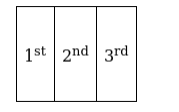# How many 3-digit numbers are there when a digit may be repeated anyQuestion:

How many 3-digit numbers are there when a digit may be repeated any numbers of time?

Solution:

Let Suppose 3 digit number as 3 boxes as shown below. First Box is at

$100^{\text {th }}$ place, the Second box is at $10^{\text {th }}$ place, and the Third box be at $1^{\text {st }}$ place.To make a 3 digit number

$1^{\text {st }}$ box can be filled with nine numbers $(1,2,3,4,5,6,7,8,9)$ if we include 0 in $1^{\text {st }}$ box then it become 2 digit number(i.e 010 is 2 digit number not 3 digit)

$2^{\text {nd }}$ box can be filled with ten numbers $(1,2,3,4,5,6,7,8,9,0)$ as repetition is allowed.

Similarly $3^{\text {rd }}$ box can be filled with ten numbers $(1,2,3,4,5,6,7,8,9,0)$

Total number of ways is $9 \times 10 \times 10=900$